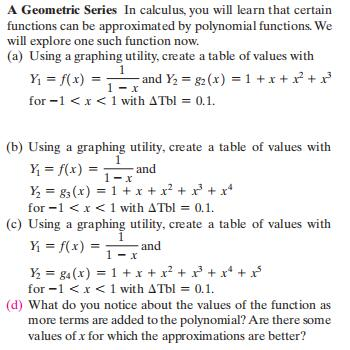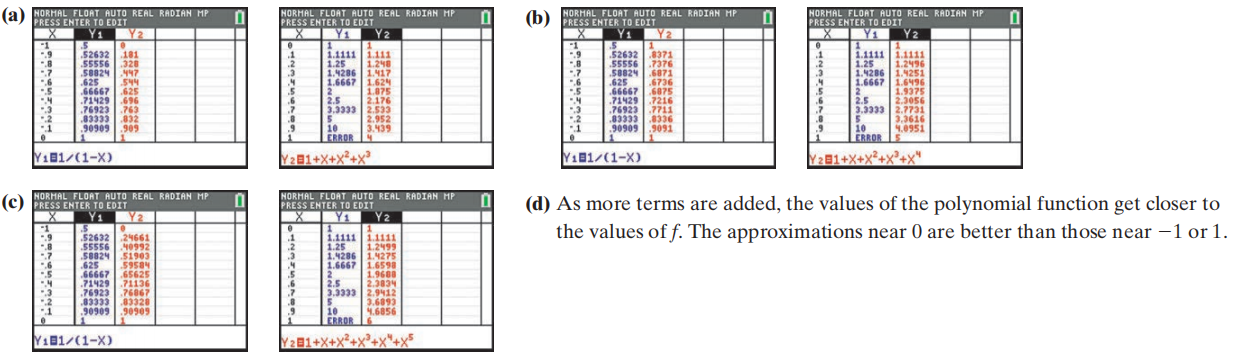### ¿Todavía tienes preguntas de matemáticas?

Pregunte a nuestros tutores expertos
Algebra
PreguntaA Geometric Series

In calculus, you will learn that certain functions can be approximated by polynomial functions. We will explore one such function now.

(a) Using a graphing utility, create a table of values with

$$Y _ { 1 } = f ( x ) = \frac { 1 } { 1 - x }$$ and $$Y _ { 2 } = g _ { 2 } ( x ) = 1 + x + x ^ { 2 } + x ^ { 3 }$$

for $$- 1 < x < 1$$ with $$\Delta$$ Tbl $$= 0.1$$ .

(b) Using a graphing utility, create a table of values with

$$Y _ { 1 } = f ( x ) = \frac { 1 } { 1 - x }$$ and

$$Y _ { 2 } = g _ { 3 } ( x ) = 1 + x + x ^ { 2 } + x ^ { 3 } + x ^ { 4 }$$

for $$- 1 < x < 1$$ with $$\Delta$$ Tbl $$= 0.1$$ .

(c) Using a graphing utility, create a table of values with

$$Y _ { 1 } = f ( x ) = \frac { 1 } { 1 - x }$$ and

$$Y _ { 2 } = g _ { 4 } ( x ) = 1 + x + x ^ { 2 } + x ^ { 3 } + x ^ { 4 } + x ^ { 5 }$$

for $$- 1 < x < 1$$ with $$\Delta$$ Tbl $$= 0.1$$ .

(d) What do you notice about the values of the function as more terms are added to the polynomial? Are there some values of $$x$$ for which the approximations are better?(d) As more terms are added, the values of the polynomial function get closer to the values of $$f$$ . The approximations near $$0$$ are better than those near $$- 1$$ or $$1$$ .